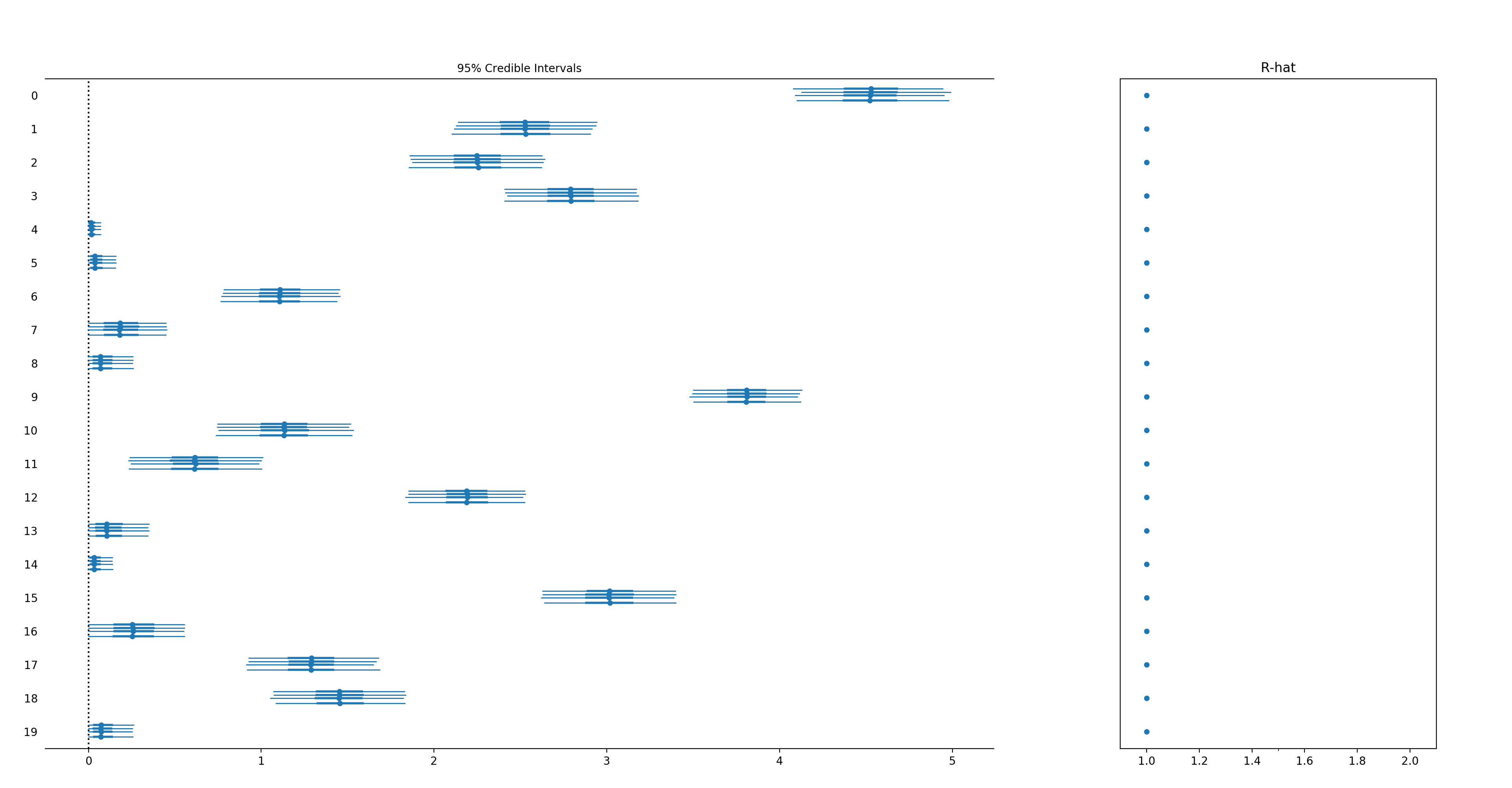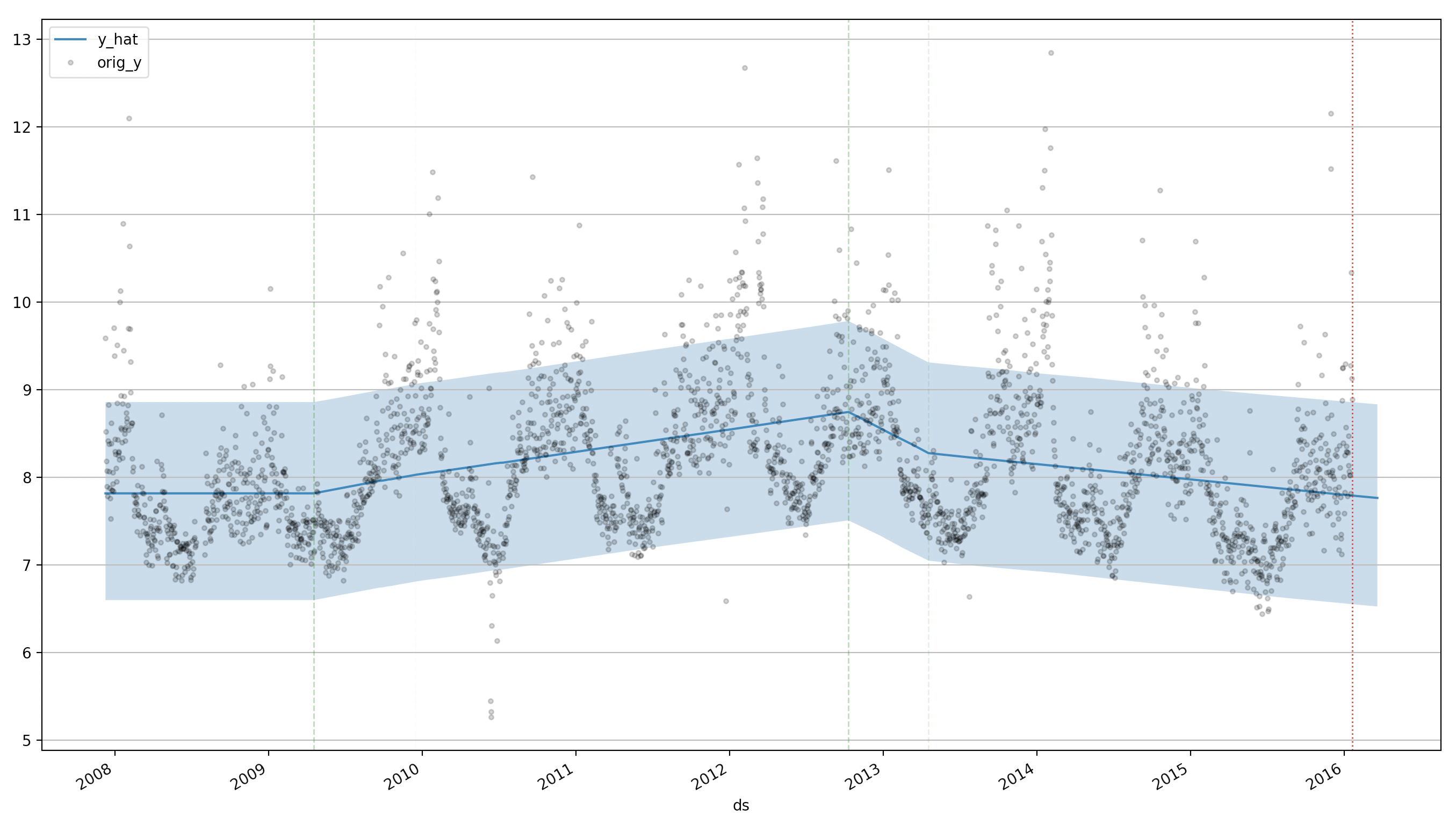Skip to content

# luke14free/pm-prophet

GAM timeseries modeling with auto-changepoint detection. Inspired by Facebook Prophet and implemented in PyMC3
Latest commit 1574ad1 Apr 8, 2019
Type Name Latest commit message Commit time
Failed to load latest commit information.examples Apr 6, 2019pmprophet Apr 8, 2019.gitignore Aug 22, 2018README.md Apr 7, 2019requirements.txt Aug 22, 2018setup.py Apr 8, 2019tests.py Apr 7, 2019

# pm-prophetPymc3-based universal time series prediction and decomposition library (inspired by Facebook Prophet). However, while Faceook prophet is a well-defined model, pm-prophet allows for total flexibility in the choice of priors and thus is potentially suited for a wider class of estimation problems.

⚠️ Only supports Python 3

## Installing pm-prophet

PM-Prophet installation is straightforward using pip: `pip install pmprophet`

Note that the key dependency of pm-prophet is PyMc3 a library that depends on Theano.

## Key Features

• Nowcasting & Forecasting
• Intercept, growth
• Regressors
• Holidays
• Additive & multiplicative seasonality
• Fitting and plotting
• Custom choice of priors (not in Facebook's prophet original model)
• Changepoints in growth
• Automatic changepoint location detection (not in Facebook's prophet original model)
• Fitting with NUTS/AVDI/Metropolis

## Experimental warning ⚠️

• Note that automatic changepoint detection is experimental

## Differences with Prophet:

• Saturating growth is not implemented
• Uncertainty estimation is different
• All components (including seasonality) need to be explicitly added to the model
• By design pm-prophet places a big emphasis on posteriors and uncertainty estimates, and therefore it won't use MAP for it's estimates.
• While Faceook prophet is a well-defined fixed model, pm-prophet allows for total flexibility in the choice of priors and thus is potentially suited for a wider class of estimation problems

## Peyton Manning example

Predicting the Peyton Manning timeseries:

```import pandas as pd
from pmprophet.model import PMProphet, Sampler

df = pd.read_csv("examples/example_wp_log_peyton_manning.csv")
df = df.head(180)

# Fit both growth and intercept
m = PMProphet(df, growth=True, intercept=True, n_changepoints=25, changepoints_prior_scale=.01, name='model')

# Add monthly seasonality (order: 3)
m.add_seasonality(seasonality=30, fourier_order=3)

# Add weekly seasonality (order: 3)
m.add_seasonality(seasonality=7, fourier_order=3)

# Fit the model (using NUTS)
m.fit(method=Sampler.NUTS)

ddf = m.predict(60, alpha=0.2, include_history=True, plot=True)
m.plot_components(
intercept=False,
)```

## Custom Priors

One of the main reason why PMProphet was built is to allow custom priors for the modeling.

The default priors are:

Variable Prior Parameters
`regressors` Laplace loc:0, scale:2.5
`holidays` Laplace loc:0, scale:2.5
`seasonality` Laplace loc:0, scale:0.05
`growth` Laplace loc:0, scale:10
`changepoints` Laplace loc:0, scale:2.5
`intercept` Normal loc:`y.mean`, scale: `2 * y.std`
`sigma` Half Cauchy tau:10

But you can change model priors by inspecting and modifying the distributions stored in

`m.priors`

which is a dictionary of {prior: pymc3-distribution}.

In the example below we will model an additive time-series by imposing a "positive coefficients" constraint by using an Exponential distribution instead of a Laplacian distribution for the regressors.

```import pandas as pd
import numpy as np
import pymc3 as pm
from pmprophet.model import PMProphet, Sampler

n_timesteps = 100
n_regressors = 20

regressors = np.random.normal(size=(n_timesteps, n_regressors))
coeffs = np.random.exponential(size=n_regressors) + np.random.normal(size=n_regressors)
# Note that min(coeffs) could be negative due to the white noise

regressors_names = [str(i) for i in range(n_regressors)]

df = pd.DataFrame()
df['y'] = np.dot(regressors, coeffs)
df['ds'] = pd.date_range('2017-01-01', periods=n_timesteps)
for idx, regressor in enumerate(regressors_names):
df[regressor] = regressors[:, idx]

m = PMProphet(df, growth=False, intercept=False, n_changepoints=0, name='model')

with m.model:
# Remember to suffix _<model-name> to the custom priors
m.priors['regressors'] = pm.Exponential('regressors_%s' % m.name, 1, shape=n_regressors)

for regressor in regressors_names:
m.add_regressor(regressor)

m.fit(
draws=10 ** 4,
method=Sampler.NUTS,
)
m.plot_components()```## Automatic changepoint detection (⚠️experimental)

Pm-prophet is equipped with a non-parametric truncated Dirichlet Process allowing it to automatically detect changepoints in the trend.

To enable it simply initialize the model with `auto_changepoints=True` as follows:

```from pmprophet.model import PMProphet, Sampler
import pandas as pd

df = pd.read_csv("examples/example_wp_log_peyton_manning.csv")
df = df.head(180)
m = PMProphet(df, auto_changepoints=True, growth=True, intercept=True, name='model')
m.fit(method=Sampler.METROPOLIS, draws=2000)
m.predict(60, alpha=0.2, include_history=True, plot=True)
m.plot_components(
intercept=False,
)```

Where n_changepoints is interpreted as the truncation point for the Dirichlet Process.

Pm-prophet will then decide which changepoint values make sense and add a custom weight to them. A call to `plot_components()` will reveal the changepoint map:A few caveats exist:

• It's slow to fit since it's a non-parametric model
• For best results use NUTS as method
• It will likely require more than the default number of draws to converge
You can’t perform that action at this time.